Maths-
General
Easy

Question

# Simplify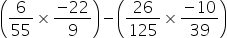## The correct answer is: rational numbers.

### To simplifyOn simplifications, we have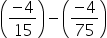Determine the LCM of the denominators of rational numbers.LCM of 15 and 75 = 75On multiplying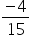with 5, we get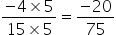On multiplying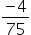with 1, we get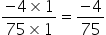On subtraction, we have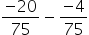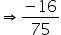Perform multiplication and then subtract.#### With Turito Foundation.#### Get an Expert Advice From Turito.